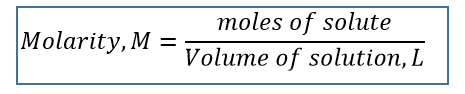# Problem: What is the molarity of a solution that is made by dissolving 3.68 g of sucrose (C12H22O11) in sufficient water to form 275.0 mL of solution?

###### FREE Expert Solution

Recall the equation for MolarityTo solve for molarity, we need to do these steps:

• Solve for molar mass of C12H22O11
• Convert the mass of solute to moles solute ( C12H22O11 )
• Convert Volume of solution from mL to L
95% (194 ratings)###### Problem Details

What is the molarity of a solution that is made by dissolving 3.68 g of sucrose (C12H22O11) in sufficient water to form 275.0 mL of solution?